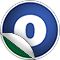# LinearCombination

### Related Docs: class LinearCombination | package linearcombination

#### object LinearCombination

Linear Supertypes
AnyRef, Any
Ordering
1. Alphabetic
2. By inheritance
Inherited
1. LinearCombination
2. AnyRef
3. Any
1. Hide All
2. Show all
Visibility
1. Public
2. All

### Value Members

1. #### final def !=(arg0: Any): Boolean

Definition Classes
AnyRef → Any
2. #### final def ##(): Int

Definition Classes
AnyRef → Any
3. #### final def ==(arg0: Any): Boolean

Definition Classes
AnyRef → Any
4. #### object Constant

Extractor applying to `LinearCombination` that are constant.

Extractor applying to `LinearCombination` that are constant.

5. #### object Difference

Extractor applying to `LinearCombination` that are the difference between two non-constant terms; with the term with positive coefficient coming first.

Extractor applying to `LinearCombination` that are the difference between two non-constant terms; with the term with positive coefficient coming first.

8. #### object SingleTerm

Extractor applying to `LinearCombination` that are just a single term with coefficient `1`.

Extractor applying to `LinearCombination` that are just a single term with coefficient `1`.

9. #### object ValueOrdering extends PartialOrdering[LinearCombination]

Ordering that relates linear combinations `a, b` if the value of `a` is always going to be in relationship with `b`.

Ordering that relates linear combinations `a, b` if the value of `a` is always going to be in relationship with `b`. E.g., ` x + 3 < x + 5 `.

16. #### def apply(terms: Iterator[(IdealInt, Term)], order: TermOrder): LinearCombination

Create a linear combination from an arbitrary set of terms with coefficients

17. #### final def asInstanceOf[T0]: T0

Definition Classes
Any
18. #### def clone(): AnyRef

Attributes
protected[java.lang]
Definition Classes
AnyRef
Annotations
@throws( ... )
19. #### def createFromSortedSeq(terms: Iterator[(IdealInt, Term)], order: TermOrder): LinearCombination

Create a linear combination from an array of coefficient-term pairs that is already sorted, flattened, and contracted.

20. #### def createFromSortedSeq(terms: Seq[(IdealInt, Term)], order: TermOrder): LinearCombination

Create a linear combination from an array of coefficient-term pairs that is already sorted, flattened, and contracted.

21. #### final def eq(arg0: AnyRef): Boolean

Definition Classes
AnyRef
22. #### def equals(arg0: Any): Boolean

Definition Classes
AnyRef → Any
23. #### def finalize(): Unit

Attributes
protected[java.lang]
Definition Classes
AnyRef
Annotations
@throws( classOf[java.lang.Throwable] )
24. #### final def getClass(): Class[_]

Definition Classes
AnyRef → Any
25. #### def hashCode(): Int

Definition Classes
AnyRef → Any
26. #### final def isInstanceOf[T0]: Boolean

Definition Classes
Any
27. #### final def ne(arg0: AnyRef): Boolean

Definition Classes
AnyRef
28. #### final def notify(): Unit

Definition Classes
AnyRef
29. #### final def notifyAll(): Unit

Definition Classes
AnyRef
30. #### def sum(coeff1: IdealInt, lc1: LinearCombination, coeff2: IdealInt, lc2: LinearCombination, coeff3: IdealInt, lc3: LinearCombination, order: TermOrder): LinearCombination

Compute the sum of exactly three linear combinations with arbitrary coefficients

31. #### def sum(coeff1: IdealInt, lc1: LinearCombination, coeff2: IdealInt, lc2: LinearCombination, order: TermOrder): LinearCombination

Compute the sum of exactly two linear combinations with arbitrary coefficients.

Compute the sum of exactly two linear combinations with arbitrary coefficients. This method is optimised and tries to avoid the the general case as far as possible

33. #### def sum(lcs: Seq[(IdealInt, LinearCombination)], order: TermOrder): LinearCombination

Compute the sum of a collection of linear combinations (together with coefficients).

Compute the sum of a collection of linear combinations (together with coefficients). This method is more optimised than direct usage of `LCBlender`

34. #### final def synchronized[T0](arg0: ⇒ T0): T0

Definition Classes
AnyRef
35. #### def toString(): String

Definition Classes
AnyRef → Any
36. #### final def wait(): Unit

Definition Classes
AnyRef
Annotations
@throws( ... )
37. #### final def wait(arg0: Long, arg1: Int): Unit

Definition Classes
AnyRef
Annotations
@throws( ... )
38. #### final def wait(arg0: Long): Unit

Definition Classes
AnyRef
Annotations
@throws( ... )